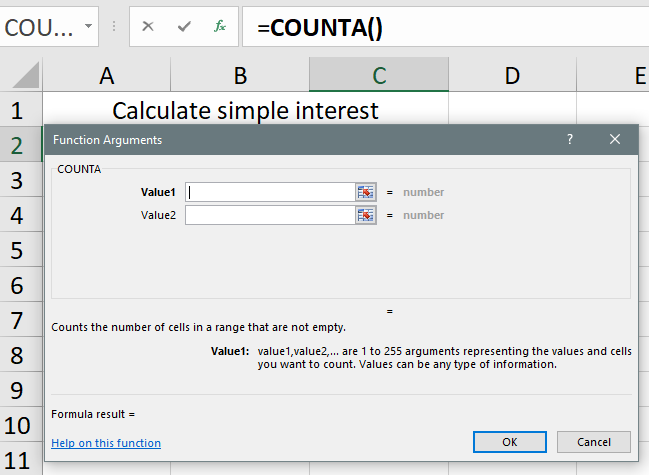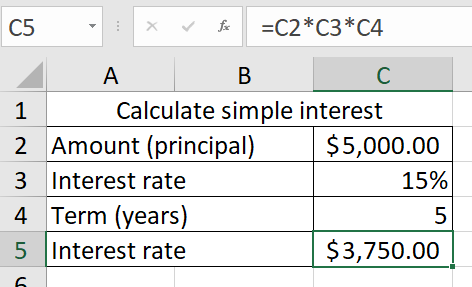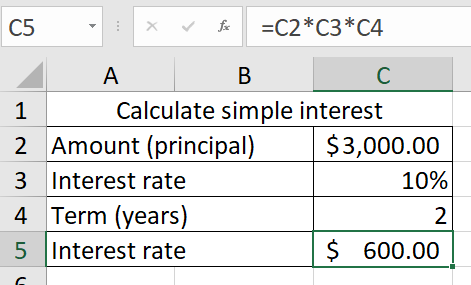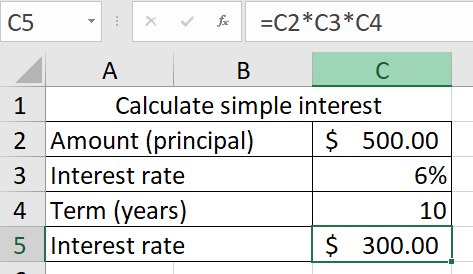Get instant live expert help with Excel or Google Sheets“My Excelchat expert helped me in less than 20 minutes, saving me what would have been 5 hours of work!”

#### Post your problem and you’ll get Expert help in seconds.

Your message must be at least 40 characters
Our professional Expert are available now. Your privacy is guaranteed.

# How to Calculate Simple Interest in Excel

There is a formula in Excel which calculates simple interest by multiplying the principal, the rate, and the term.

## Calculate simple interest in Excel

Consider the example demonstrated below in which the formula in C5 is =C2*C3*C4

In order to do a simple interest calculation in Excel using the COUNTA function, follow the procedure below:

• Click on Formulas on the menu bar in Excel.
• Next, click on More Functions and point the cursor on Statistical.
• Scroll down the list that displays and click on COUNTA.

The dialogue box will display as shown below.Figure 1. How to access COUNTA formula in Excel

### Example 1

• The value 1 represents the span of cells to which you want to apply the formula. In the example below, it is C2 to C4. Type C2:C4.
• Click on OK.

It will show you the result displayed in the screenshot below:Figure 2. The result displayed by COUNTA

### Interpretation of the Result

What is displayed above shows that for a given amount of money (principal), say \$5000, invested at the interest rate of 5 percent per year for 15 years, using the COUNTA function to calculate the interest generated, that is, =C2*C3*C4 the answer is \$3,750. It is possible to verify this formula by manually doing the calculation as shown below:

Interest = Amount X Rate X Term

= 5000X0.05X15

= 3,750.

Alternatively, you can still calculate the simple interest by simply typing the formula above into the cell on the right of the row you are interested in. Hit the enter key when you finish typing, and the result will show.

### The General Formula

The general formula for calculating simple interest in Excel is shown below:

Interest = Principal*Rate*Term

This means that you have to multiply the principal by the rate and by the term.

In the example demonstrated above, the amount of \$5000 is invested at the rate of 5% per annum for a period of 15 years. The formula imputed into C5 is

`=C2*C3*C4`

### Example 2

Calculate the simple interest on \$3000 invested at the rate of 10% per annum for 2 years using Excel.Figure 3. Simple interest on \$3000 invested at the rate of 10% per annum for 2 years

The same principle applies. In this example, the formula in C5 is

`=C2*C3*C4`

It can also be verified by doing the calculation physically as shown below:

Interest = Amount X Rate X Years

= 3000 X 10 X 2

= 600.

### Example 3

Calculate the simple interest on \$500 invested at the rate of 6% per annum for 10 years using Excel.Figure 4. Simple interest on \$500 invested at the rate of 6% per annum for 10 years

In the example above, the formula in C5 is

`=C2*C3*C4`

However, because the values are different, the interest calculated is different also. This too can also be verified by doing the calculation physically as shown below:

Interest = Amount X Rate X Years

= 500 X 6 X 10

= 300.

Still need some help with Excel formatting or have other questions about Excel? Connect with a live Excel expert here for some 1 on 1 help. Your first session is always free.

### Did this post not answer your question? Get a solution from connecting with the expert.Another blog reader asked this question today on Excelchat:
Solution examplesI have a Heading Title at the top of each column and keep my tally (formula) of that column in the second row. However, ever time I sort/filter my worksheet the second row also gets sorted and I lost my tally - which I use for analysis in the second sheet. I have tried freeze and split and neither works. Please help !
Solved by F. Q. in 40 minsHi, Hopefully someone will be able to help me. I'm wanting to calculate the average sum contained within a range of cells discarding blanks. The problem that I'm encountering is that there is an additional feature where I only want to take into consideration columns that contain specific text. =SUMIF(G79:HG79,"Sep-18",(G6:HG6/COUNTA(G6:HG6))) G79:HG79 will contain the specific text with G:HG containing the digits. Cheers all in advance
Solved by M. J. in 20 mins## Subscribe to Excelchat.coAnother blog reader asked this question today on Excelchat: